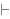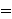Intuitionistic Logic Explorer < Previous   Next > Nearby theorems Mirrors  >  Home  >  ILE Home  >  Th. List  >  a9e Unicode version

Theorem a9e 1627
 Description: At least one individual exists. This is not a theorem of free logic, which is sound in empty domains. For such a logic, we would add this theorem as an axiom of set theory (Axiom 0 of [Kunen] p. 10). In the system consisting of ax-5 1377 through ax-14 1446 and ax-17 1460, all axioms other than ax-9 1465 are believed to be theorems of free logic, although the system without ax-9 1465 is probably not complete in free logic. (Contributed by NM, 5-Aug-1993.) (Revised by NM, 3-Feb-2015.)
Assertion
Ref Expression
a9eProof of Theorem a9e
StepHypRef Expression
1 ax-i9 1464 1Colors of variables: wff set class Syntax hints:wex 1422 This theorem was proved from axioms:  ax-i9 1464 This theorem is referenced by:  ax9o  1629  equid  1630  equs4  1654  equsal  1656  equsex  1657  equsexd  1658  spimt  1665  spimeh  1668  spimed  1669  equvini  1682  ax11v2  1742  ax11v  1749  ax11ev  1750  equs5or  1752  euequ1  2037
 Copyright terms: Public domain W3C validator